# James Williams

## Fun with Fractals and Flame

#### Tags: game programmingflutterflame

Fractals are patterns that are self-similar across scale, meaning a part of the object is similar to the whole. Their earliest applications revolved around simple recursion but today there is a whole field of study for generating fractals and the search to find formulas that will replicate nature. Fractals exist in many parts of nature and biology including trees, DNA, blood vessels, lighting, and some foods.

Before the first computer generated fractals were created, man-made fractals have been noticed in ancient and modern architecture and textiles. In game development, procedural generation of fractals or cellular automata (like Conway's Game of Life) are simple ways to provide everything needed for a game loop without needing a more defined game concept.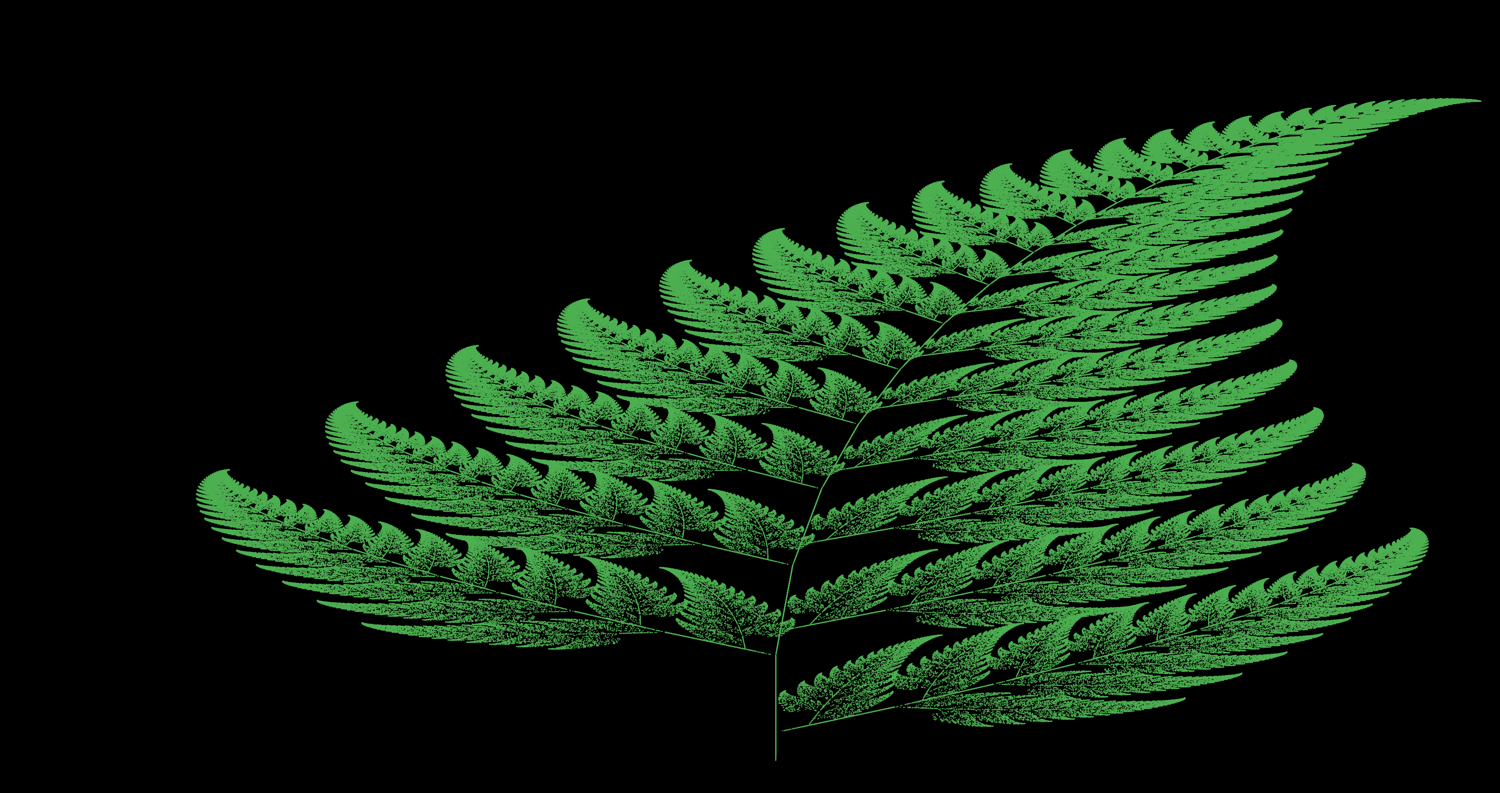## Sierpinski Carpet

A Sierpinski carpet attains its self-similarity but subdividing itself into a number of copies of the whole, removes one, and recurses on the subdivided copies. One formula to create a Sierpinski carpet is to:

2. Subdivide it into 9 squares (3x3)
3. Delete the center square.
4. Repeat.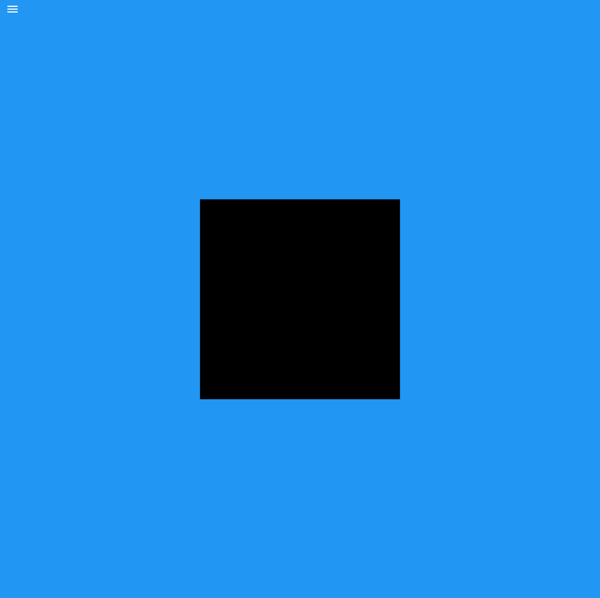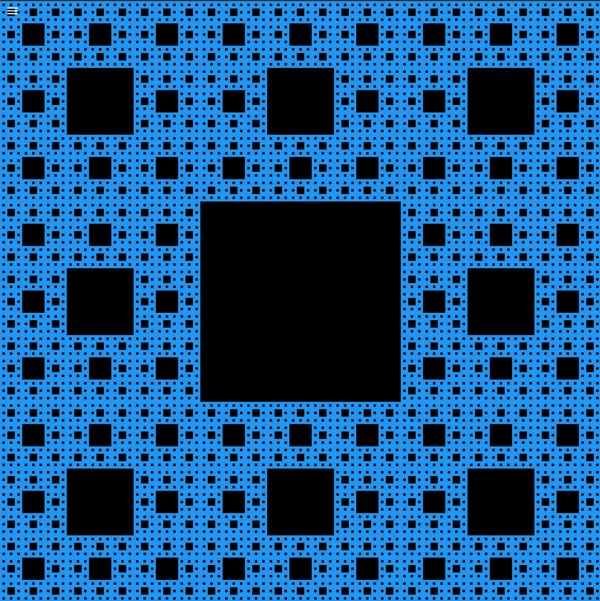``````import 'package:flutter/material.dart';
import 'package:flame/game.dart';
import 'package:flame/components.dart';

class SierpinskiCarpet extends FlameGame {
late Size dimens;
final RECURSIONS = 5;
var white = Paint()..color = Colors.white;
var black = Paint()..color = Colors.black;
var blue = Paint()..color = Colors.blue;

double shortestSide() {
return dimens.width < dimens.height ? dimens.width : dimens.height;
}
@override
dimens = canvasSize.toSize();
double square = shortestSide();
var bounds = RectangleComponent(position:Vector2(0,0), size:Vector2.all(square), paint:blue);

// Draw a white square matching the bounds

punchCantorGasket(bounds.position.x, bounds.position.y, bounds.size.x, RECURSIONS);
}
void punchCantorGasket( double x, double y, double size, int recursions) {
// Base case, if recursions = 0, return
if (recursions == 0) {
return;
}

double newSize = size / 3.0;
double newSize2 = newSize * 2;
var newRect = RectangleComponent(position: Vector2(x+newSize, y + newSize), size:Vector2.all(newSize), paint: black);

recursions--;

// Call punchCantorGasket on all 8 other squares
punchCantorGasket(x, y, newSize, recursions); // 0,0
punchCantorGasket(x, y + newSize, newSize, recursions); // 0,1
punchCantorGasket(x, y + newSize2, newSize, recursions); // 0,2

punchCantorGasket(x + newSize, y, newSize, recursions); // 1,0
punchCantorGasket(x + newSize, y + newSize2, newSize, recursions); // 1, 2

punchCantorGasket(x + newSize2, y, newSize, recursions); // 2,0
punchCantorGasket(x + newSize2, y + newSize, newSize, recursions); // 2,1
punchCantorGasket(x + newSize2, y + newSize2, newSize, recursions); // 2,2
}
}
``````

## Barnsley Fern

Iterated function systems (IFS) create fractals by taking copies of itself, mutating the copy in some way and then unioned with the rest of the system. First described in the book Fractals Everywhere by its namesake Michael Barnsley, the Barnsley Fern simulates black spleenwort (Asplenium adiantum-nigrum).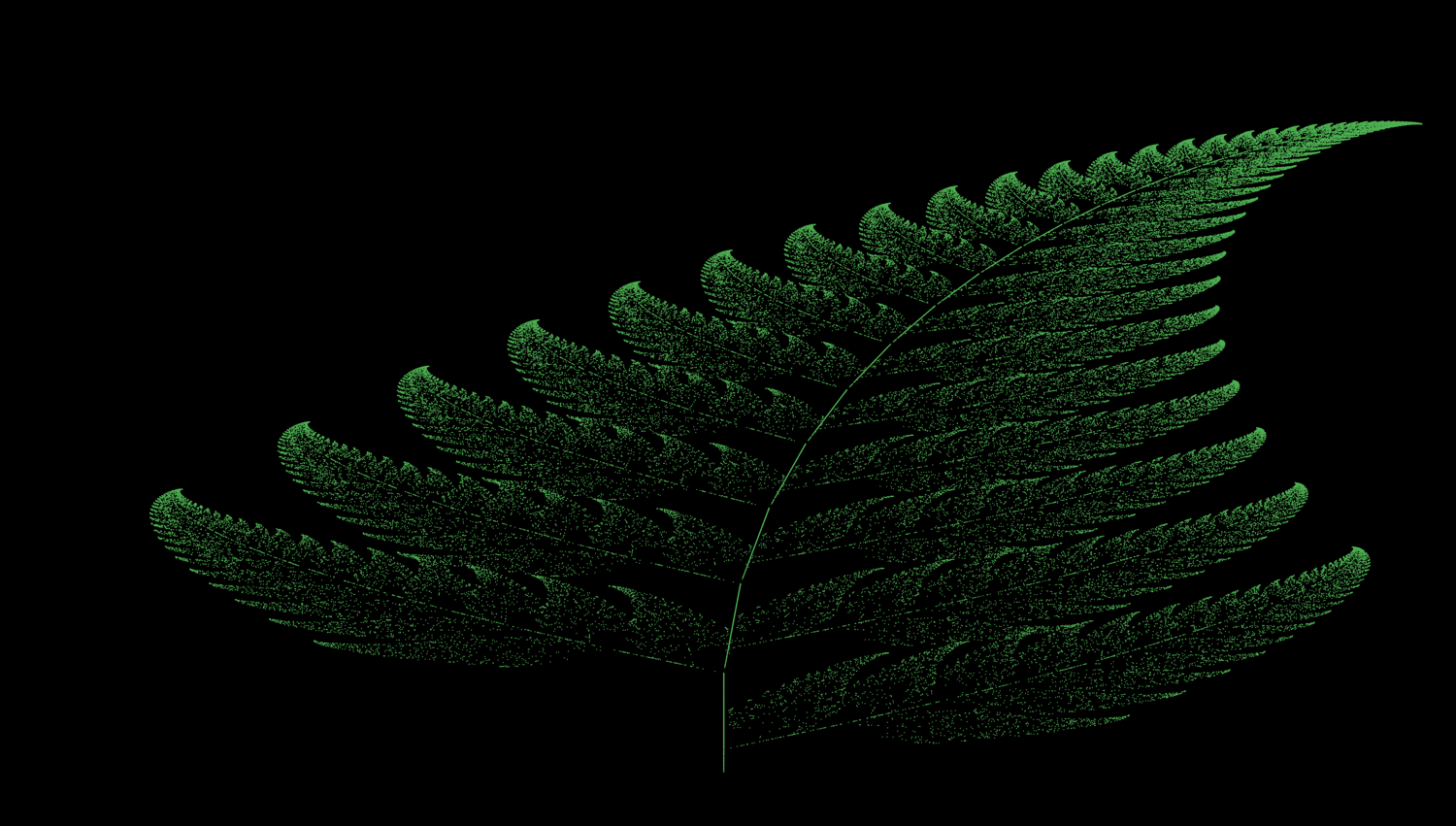The IFS uses 4 transformations each with different probability frequencies shown in the table below.

 w a b c d e f p Portion Generated ƒ1 0 0 0 0.16 0 0 0.01 Stem ƒ2 0.85 0.04 -0.04 0.85 0 1.6 0.85 Smaller leaflets ƒ3 0.20 -0.26 0.23 0.22 0 1.6 0.07 Largest left-hand leaflet ƒ4 -0.15 0.28 0.26 0.24 0 0.44 0.07 Largest right-hand leaflet
The starting point of the system is set to (0,0). The next point is created by calculating a random double and using its value to determine which transformation to apply with the last x and y coordinates resulting in the new location to plot. As more points are plotted, the form of the fern takes shape.
``````import 'package:flame/components.dart';
import 'package:flame/game.dart';
import 'package:flutter/material.dart';
import 'dart:math';
import 'dart:async' as Async;

class BarsleyFern extends FlameGame {
final RADIUS = 0.6;

final interval = Duration(milliseconds: 200);

Random rnd = Random();
Paint leafPaint = Paint()..color = Colors.green;
Vector2 currentPoint = Vector2.all(0.0);

@override
Future onLoad() async {
// Flame provides its own timer, and we want to use the core Flutter one.
Async.Timer.periodic(interval, drawLeaves);
}

void drawLeaves(Async.Timer t) {
for (var i = 0; i < 500; i++) {
currentPoint = calculateNextPoint(currentPoint);
drawCircle(currentPoint);
}
}

Vector2 calculateNextPoint(Vector2 lastPoint) {
var r = rnd.nextDouble();
Vector2 nextPoint = Vector2.all(0.0);
if (r < 0.01) {
nextPoint.x = 0;
nextPoint.y = 0.16 * lastPoint.y;
}
else if (r < 0.86) {
nextPoint.x = 0.85 * lastPoint.x + 0.04 * lastPoint.y;
nextPoint.y = -0.04 * lastPoint.x + 0.85 * lastPoint.y + 1.6;
}
else if (r < 0.93) {
nextPoint.x = 0.20 * lastPoint.x - 0.26 * lastPoint.y;
nextPoint.y = 0.23 * lastPoint.x + 0.22 * lastPoint.y + 1.6;
}
else {
nextPoint.x = -0.15 * lastPoint.x + 0.28 * lastPoint.y;
nextPoint.y = 0.26 * lastPoint.x + 0.24 * lastPoint.y + 0.44;
}

return nextPoint;
}

void drawCircle(Vector2 point) {
// Scale point to canvas
Vector2 plot = Vector2.all(0.0);
plot.x = canvasSize.x * (point.x + 3) / 6;
plot.y = canvasSize.y - (canvasSize.y * (point.y + 2)/14);
}
}
``````
This animation draws 500 points every 200 milliseconds.

## Dragon Curve

A Lindenmayer system (L-system) uses an alphabet with production rules to expand the resulting string. Encoded into the alphabet are in instructions like moving a distance, rotating by an angle, or a combination of these rules. Aristid Lindenmayer, the theoretical botanist and biologist for whom they are named, used them to describe the growth of simple organisms such as bacteria. L-systems can be seen in herbaceous plants and trees. His work survives in a posthumously published book, The Algorithmic Beauty of Plants. The Dragon Curve is a fractal made by a single line naively by repeatedly folding each edge towards 90 degree angles.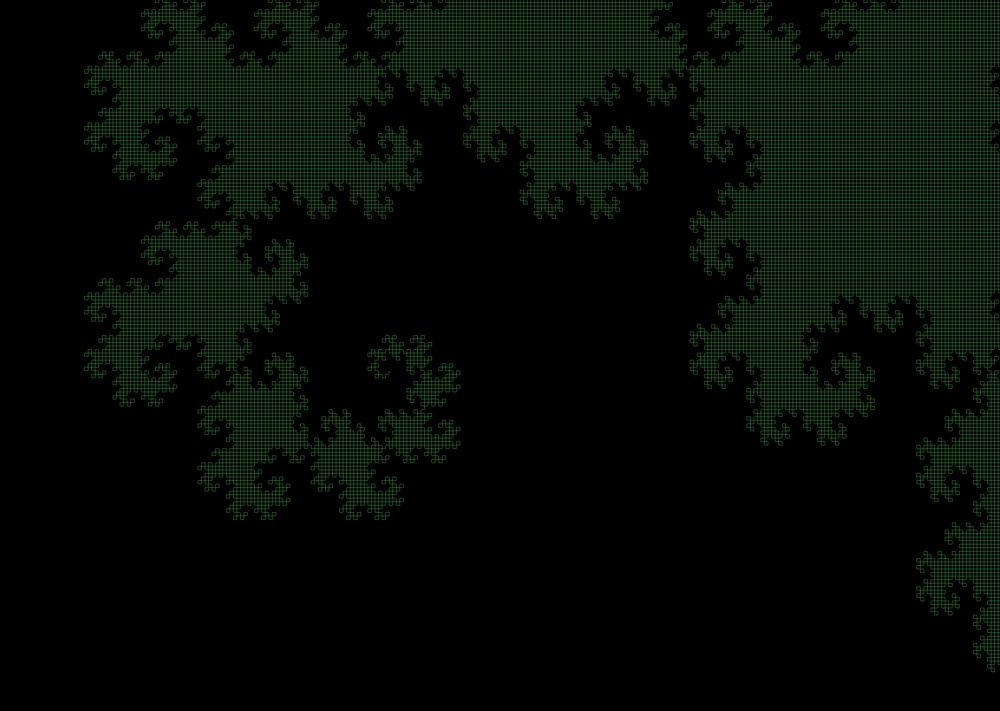``````Given the grammar:
Variables: F G

Constants: + -

Start: F
Angle: 90

Rules:
F -> F+G
G -> F-G
``````
F and G are instructions to draw forward by a scalar amount. + means turn left by angle and - means turn right by that same angle. In the case of dragon curves, you don't need to recursively expand the string using the production rules. An alternate way of producing a dragon curve is to reduce the grammar to draw forward and do a left or right turn at the same time (L and R). You can now make a dragon curve by taking the output of the previous curve, adding a left turn and appending the result of reversing and inverting the previous curve.
 Step Expansion 0 L 1 L + L + R 2 LLR + L + LRR 3 LLRLLRR + L + LLRRLR
``````import 'package:flutter/material.dart';
import 'package:flame/game.dart';
import '../new_shape_components/line.dart';

/*
The Dragon Curve is a fractal made by a single line. It is formed of a series of turns, which can be constructed in the following way:
0: L
1: L + L + R
2: LLR + L + LRR
3: LLRLLRR + L + LLRRLRR
The nth dragon curve is the n-1th dragon curve plus L, plus the n-1th dragon curve reversed and reflected.
In this project we have split up the tasks of generating and drawing the dragon curve into separate classes.
*/

class DragonCurve extends FlameGame{
final RECURSIONS = 15;

@override
Future onLoad() async {
List dragonCurve = DragonCurveGenerator().generateDragonCurve(canvasSize.x.toInt(), canvasSize.y.toInt(), RECURSIONS);
add(Polyline(dragonCurve, Paint()..color = Colors.green));
}
}
enum Direction { LEFT, RIGHT}

class DragonCurveGenerator {
Vector2 turn(Vector2 heading, Direction turn) {
var newHeading = Vector2.all(0.0);
if (turn == Direction.LEFT) {
} else {
}
}

List dragonTurns(int recursions) {
List turns = [];

for(int i = 0; i < recursions; i++) {
// Add a left turn to turns
// Add reflected version of reversed to turns
for(int j = turns.length-2; j >= 0; j--) {
if (turns[j] == Direction.LEFT)
}
}
return turns;
}

List generateDragonCurve(int width, int height, int recursions) {
var turns = dragonTurns(recursions);

var head = Vector2(width/2, height/2);
var heading = Vector2(5, 0);

var curve = [];